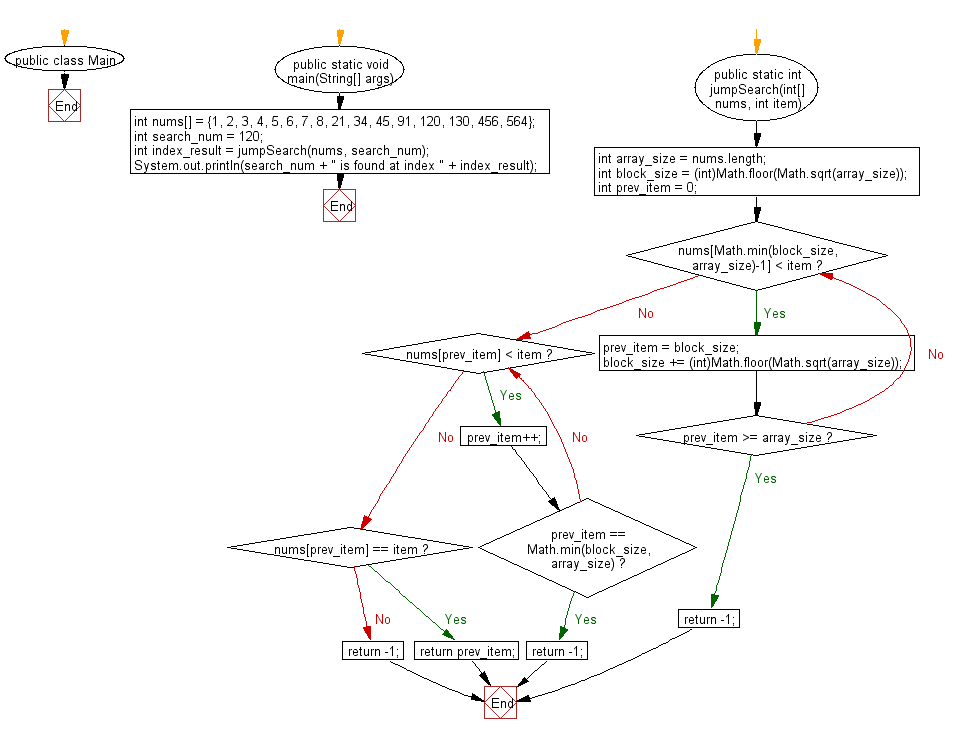﻿ Java exercises: Find a specified element in a given sorted array of elements using Jump Search - w3resource# Java Exercises: Find a specified element in a given sorted array of elements using Jump Search

## Java Search: Exercise-3 with Solution

Write a Java program to find a specified element in a given sorted array of elements using Jump Search.

From Wikipedia, in computer science, a jump search or block search refers to a search algorithm for ordered lists. It works by first checking all items Lkm, where ℜ ∈ ℵ and m is the block size, until an item is found that is larger than the search key. To find the exact position of the search key in the list a linear search is performed on the sublist L[(k-1)m, km].
Algorithm:
Input: An ordered list L, its length n and a search key s.
Output: The position of s in L, or nothing if s is not in L.

```  a ← 0
b ← [√n]

while Lmin(b,n)-1 < s do
a ← b
b ← b + [√n]
if a ≥ n then
return nothing

while La < s do
a ← a + 1
if a = min(b,n)
return nothing

if La = s then
return a
else
return nothing
```

Sample Solution:

Java Code:

``````public class Main {

public static void main(String[] args) {
int nums[] = {1, 2, 3, 4, 5, 6, 7, 8, 21, 34, 45, 91, 120, 130, 456, 564};
int search_num = 120;

// Find the index of searched item
int index_result = jumpSearch(nums, search_num);

System.out.println(search_num + " is found at index " + index_result);

}

public static int jumpSearch(int[] nums, int item)	    {

int array_size = nums.length;

// Find block size to be jumped
int block_size = (int)Math.floor(Math.sqrt(array_size));

// If the element is present find the block where element is present
int prev_item = 0;
while (nums[Math.min(block_size, array_size)-1] < item)
{
prev_item = block_size;
block_size += (int)Math.floor(Math.sqrt(array_size));
if (prev_item >= array_size)
return -1;
}

// Using a linear search for element in block beginning with previous item
while (nums[prev_item] < item)
{
prev_item++;
if (prev_item == Math.min(block_size, array_size))
return -1;
}

// If element is found
if (nums[prev_item] == item)
return prev_item;

return -1;
}
}
```
```

Sample Output:

```120 is found at index 12
```

Flowchart:Java Code Editor:

What is the difficulty level of this exercise?

﻿

## Java: Tips of the Day

Array vs ArrayLists:

The main difference between these two is that an Array is of fixed size so once you have created an Array you cannot change it but the ArrayList is not of fixed size. You can create instances of ArrayLists without specifying its size. So if you create such instances of an ArrayList without specifying its size Java will create an instance of an ArrayList of default size.

Once an ArrayList is full it re-sizes itself. In fact, an ArrayList is internally supported by an array. So when an ArrayList is resized it will slow down its performance a bit as the contents of the old Array must be copied to a new Array.

At the same time, it's compulsory to specify the size of an Array directly or indirectly while creating it. And also Arrays can store both primitives and objects while ArrayLists only can store objects.

Ref: https://bit.ly/3o8L2KH